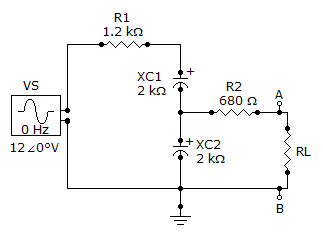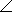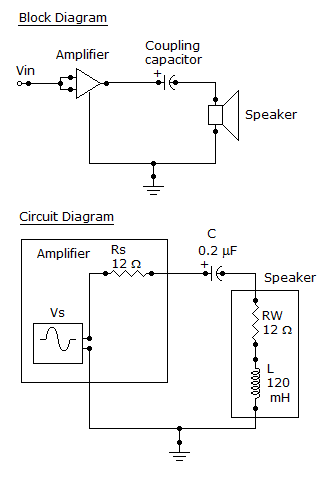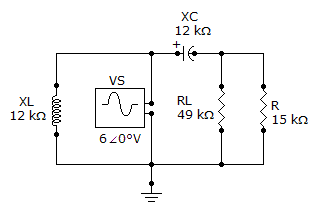# Electrical Engineering - Circuit Theorems in AC Analysis

### Exercise :: Circuit Theorems in AC Analysis - General Questions

16.

Referring to the given circuit, determine ZTH as seen by RL.A. 1444–48.5°B. 4176–73.3°C. 956–48.5°D. 1444–73.3°Explanation:

No answer description available for this question. Let us discuss.

17.

Referring to the given circuit, how much power, in watts, is delivered to the speaker at the determined frequency if VS = 4.5 VRMS?A. 226 mW B. 2.26 mW C. 4.24 mW D. 424 mW

Explanation:

No answer description available for this question. Let us discuss.

18.

The Norton equivalent current is

 A. the current through the load B. the open-current from the source C. the short circuit current D. none of the above

Explanation:

No answer description available for this question. Let us discuss.

19.

Determine VTH if R1 is changed to 3.3 k.A. 0.57416.7° V B. 4.6316.7° V C. 4.6339.5° V D. 0.46339.5° V

Explanation:

No answer description available for this question. Let us discuss.

20.

Referring to the given circuit, what is VTH if VS = 120° V?A. 4.6938.7° V B. 9.3838.7° V C. 120° V D. 60° V